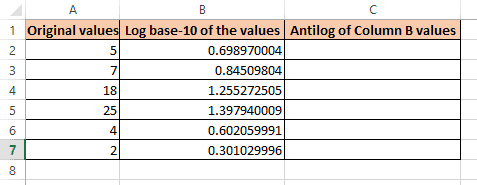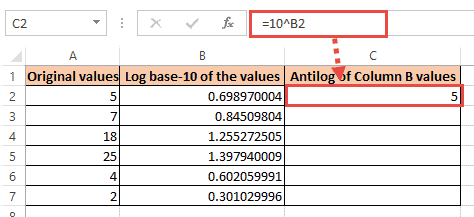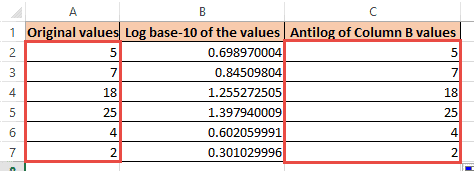# Calculate Antilog in Excel [ Easy Formula to Use ]When dealing with data that has exponential values, Logarithmic functions are frequently used.

When working with log functions, you are going to need to find the antilog at some point.

If you are working in Excel, you have most likely noticed that it does not have a function to find the antilog value.

If you understand the basics of logs and antilogs, this workaround should be intuitive and easy to understand;

Let’s first review the basic math concepts behind logs and antilogs, then we will work through how to quickly find the antilog of any number using Excel.

## What the Log of a Number Tells You

To put it simply, the log of a number is the power to which a base of 10 must be raised .

The base of the logarithm is the factor that is being repeatedly multiplied.

Let’s look at an example:

Let’s find the log of the number 1000 to the base of 10.

We are trying to find how many times the number 10 goes into the number 1,000.

In other words, how many times does 10 need to be multiplied to get to 1,000?

Here is how you find the the log of 1,000 to the base of 10:

10 x 10 x 10 = 1,000

The log to the base of 10 of 1,000 is 3 because we needed to multiply it 3 times to get to 1,000.

If you want to express this in a formula, it would look like this:

`Log10(1000) = 3`

Logarithms are used to express large numbers as a single digit number.

As we saw in the example above, 1,000 was expressed as a single-digit number, 3.

Let’s look at another example:

Let’s find the log of the number 100,000 with the number 10:

10 x 10 x 10 x 10 x 10 x 10 = 100,000

The log to the base of 10 of 100,000 is 6 because we needed to multiply it 6 times to get to 100,000.

If we were to find the log of these same numbers in excel, we would use the following formula in any given cell:

=LOG10(1000)

The result should be 3

or

=LOG10(100,000)

The result should be 6

You can also use the LOG function to specify your base:

=LOG(81,3)

The result should be 3 (3 x 4 = 81).

## Log and Antilog – what is the Relationship?

The inverse of the log of a number is the antilog number (to the base of 10).

Finding the antilog number is quite easy, you simply need to raise it to the power of 10.

Let’s look an example:

The base-10 log of the number 5 is 0.69897.

`100.69897 = 5`

The formula used to find the antilog is:

LOG10(x) = a

Antilog “a” would be:

`x = 10a`

## Calculating Antilog using Excel

Now that we have a basic understanding of log and antilog, we can review how to find the values in Excel.

As a reminder, finding the antilog of a number base-10 in Excel simply means finding the value of 10 raised to the power of that number.

For this, we use the exponent operator (‘^’).

So 105 is written as 10 ^ 5.

Let us say you have the following list of values (column A) and their logs (column B).Here are the steps needed to find the antilogs values of column B:

1. Select the first cell where you want the result to be displayed. In our example, select cell C2.
2. Enter the formula: =10^B2
3. Press the Enter or Return key.
4. This will display the antilog of the value B2 in cell C2.5. Copy this formula to the rest of the cells of column C by dragging down the fill handle. This will give you the antilog numbers for each cell.Note that the Original Values in column A are the same as the Antilog values in Column B.

This is a great way to double check that your formulas are correct.

Denise is a Financial Controller with experience in Office Software (Excel & Word), Accounting, Bookkeeping, Business Incorporation/LLC, Taxes and IRS Issues and more. She Graduated from Portland State University in Business Management and went onto Seattle University to complete her Graduate (Masters) Degree in Organizational Systems Renewal. She contributes to Computing.net with her knowledge of Business Software, in particularly Microsoft Excel and Google Sheets.